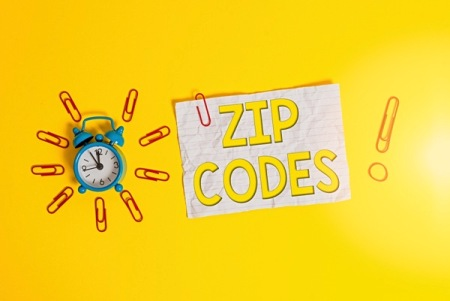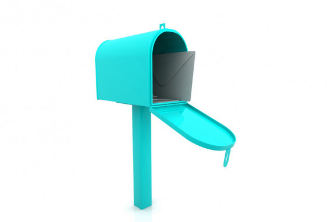﻿

# Newton, MS Zip Code

Find zip code of address in Newton, MS on the map. Find zip code by address or by point doing click on the map. Drag to change point.

Zip code:

Full screen

Newton information

ZIP code: 39345

Area code(s): 601

Population: 3373

Elevation: 427m (1401ft)

County: NewtonPostcode area of Newton is 39345.

Area code(s) of Newton is 601.

Newton has 427m (1401ft) altitude.

The coordinates of Newton are: 32.32500 lat, -89.15944 lng.

Newton belongs to Newton County.

*sources: wikipedia.org